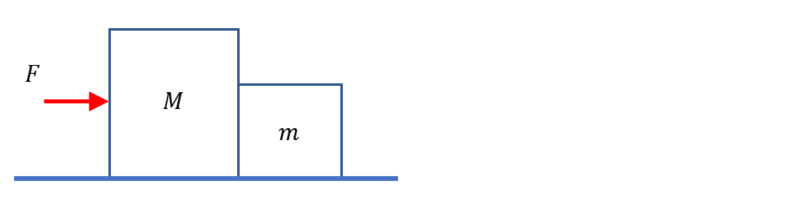# Problem: Two boxes, one of mass M and one of mass m, lie next to each other. If a force F is applied on box M, as indicated in the figure, draw a free body diagram for each box, with the appropriate value for each force given in terms of known vairables (M, m, F and g). Assume there is no friction along the surface.

###### Problem Details

Two boxes, one of mass M and one of mass m, lie next to each other. If a force F is applied on box M, as indicated in the figure, draw a free body diagram for each box, with the appropriate value for each force given in terms of known vairables (M, m, F and g). Assume there is no friction along the surface.